﻿ The Effect of Zr Concentration on Structural, Optical and Electrical Properties of Pb(Zr<SUB>x</SUB>,Ti<SUB>1-x</SUB>)O<SUB>3</SUB> PZT Prepared by Pulsed Laser Deposition Technique

### The Effect of Zr Concentration on Structural, Optical and Electrical Properties of Pb(Zrx...

Ghuson H. Mohammed, Abdul Kareem D. Ali, Hameed A. Radwan

International Journal of Physics

## The Effect of Zr Concentration on Structural, Optical and Electrical Properties of Pb(Zrx,Ti1-x)O3 PZT Prepared by Pulsed Laser Deposition Technique

Ghuson H. Mohammed1, Abdul Kareem D. Ali2, Hameed A. Radwan2,1Department of Physics, University of Baghdad / College of Sciences

2Department of Physics, University of Tikrit/College of Education

### Abstract

Pb(Zrx,Ti1-x)O3 PZT thin films with various ratio of Zr / Ti (x=0.1, 0.3, 0.5, 0.7, 0.9) deposited on glass by pulsed laser deposition technique to investigate the structural, optical and electrical properties in these films. The films were deposited at room temperature. X-ray diffraction (XRD) analysis for PZT illustrated a perovskite phase with a polycrystalline structure at RT for all samples. Optical studies showed that the optical energy gap increases with increasing Zr concentration. The d.c. conductivity (σd.c) decreased with increasing of Zr content. It was observed that the films have two activation energies that decrease with the increase of Zr content. Hall measurements showed that all the films were p-type and the carriers concentration were increase with the increasing of Zr, the mobility inversely proportional with the carriers concentration.

• Ghuson H. Mohammed, Abdul Kareem D. Ali, Hameed A. Radwan. The Effect of Zr Concentration on Structural, Optical and Electrical Properties of Pb(Zrx,Ti1-x)O3 PZT Prepared by Pulsed Laser Deposition Technique. International Journal of Physics. Vol. 5, No. 1, 2017, pp 30-36. http://pubs.sciepub.com/ijp/5/1/5
• Mohammed, Ghuson H., Abdul Kareem D. Ali, and Hameed A. Radwan. "The Effect of Zr Concentration on Structural, Optical and Electrical Properties of Pb(Zrx,Ti1-x)O3 PZT Prepared by Pulsed Laser Deposition Technique." International Journal of Physics 5.1 (2017): 30-36.
• Mohammed, G. H. , Ali, A. K. D. , & Radwan, H. A. (2017). The Effect of Zr Concentration on Structural, Optical and Electrical Properties of Pb(Zrx,Ti1-x)O3 PZT Prepared by Pulsed Laser Deposition Technique. International Journal of Physics, 5(1), 30-36.
• Mohammed, Ghuson H., Abdul Kareem D. Ali, and Hameed A. Radwan. "The Effect of Zr Concentration on Structural, Optical and Electrical Properties of Pb(Zrx,Ti1-x)O3 PZT Prepared by Pulsed Laser Deposition Technique." International Journal of Physics 5, no. 1 (2017): 30-36.

 Import into BibTeX Import into EndNote Import into RefMan Import into RefWorks

1234
Prev Next

### 1. Introduction

Discovery Semiconductors is one of the great scientific and technological advances of the 20th century, this has caused major economic change and may change our civilization . Ferroelectric ceramic, dielectric hysteresis and non-linear behavior has been of interest since the 1950s, when these substances are found in the applications of various electronic devices . An applications with a high range of user areas require future devices with more reduced size and power exhaustion, very-high speed, and high levels of implementation. To meet these challenges, we are in need to develop novel materials with improved qualities .

Pb(Zrx,Ti1-x)O3 perovskite oxide is an attractive material due to its physical properties such as Ferroelectricity, piezoelectricity and high dielectric constant. These characteristics make PZT thin films are useful in a various applications such as microelectromechanical systems, magnetoelectric devices [4, 5], fuel injection system in automobile industry, aerospace vibration control, energy harvesting from different vibration sources , sensors, infrared detectors,  and so on.

Different techniques are used to prepared PZT thin films [8, 9], including both chemical and physical routes, such as plasma enhanced chemical vapor deposition, metal-organic chemical vapor deposition, sol-gel processing, evaporation, pulsed laser deposition and sputtering deposition, however, the properties of thin films relay to some extent on the method of fabrication [9, 10]. The most important deposition technique is PLD due its ability to transfer material compatibility from a multicomponent target to a growing film. PLD a promising technique for making thick film because it is offers the advantage of a high deposition rate. Because of High deposition rate in pulses, in addition to, mass transfer column erosion of solid thin films is improved. This allows sediment in the high growth temperatures, especially for epitaxial growth of volatiles [10, 11]. The aim of the present work is to fabricate and analyze the thin films structural and morphological characteristics of Pb(Zrx,Ti1-x)O3 using PLD processing method.

### 2. Experimental Procedure

Bulk samples of Pb(Zrx,Ti1-x)O3 have been prepared by solid state reaction process. The powder of Lead dioxide, Zirconium dioxide and Titanium dioxide with a purity of 99.99% were Grinded and mixed together at a different concentration of (x = 0.1, 0.3, 0.5, 0.7, 0.9) wt. % of the formula Pb(Zrx,Ti1-x)O3 in a mixture machine for (10 minute). After that it was pressed into pellets with (1.2 cm) diameter and (0.2 cm) thick, using hydraulic piston type (SPECAC), under the pressure of 6 tons/cm2 for 10 minutes. The pellets were sintered in air to temperature (1073 K) for 2 hours then cooled to room temperature. The temperature of the furnace was raised at a rate of 250°C/hour.

The substrate used in this work for deposit of Pb(Zrx,Ti1-x)O3 thin films is the glass slides made in china from " AFCO", with dimensions (75 × 25 × 1.2 mm). The substrate cleaned in the following steps:

1-The substrates were cleaned in distilled water to remove the impurities and residual Grime from the their surface.

2-The substrates were cleaned in alcohol by an ultrasonic system for 15 min in order to remove grease and some oxides, and dried by blowing air.

3-Eventually, The slides were wiped with soft paper.

The pulsed laser deposition experiment is fulfilled inside a vacuum chamber under (10-3 mbar) vacuum conditions. The focused Nd:YAG laser beam at 800 mJ with a frequency second radiation at 1064 nm (pulse width 9 ns) frequency (6 Hz), for 400 laser pulses incident on the target surface makes an angle of 45° with it. The distance between the target and the laser gun is set to 15 cm, and between the target and the substrate is (2cm).

a. X-ray Diffraction analysis:

The structure of Pb(Zrx,Ti1-x)O3 alloys as mass and film by X-ray diffraction using a Philips X-ray diffraction system that records the intensity as a function of Bragg angle is investigated. The wavelength of the radiation source Cu Kα = 1.5405 Å, 20 mA current and the voltage was 30 kV. 2θ scan angle in the range (20-70) degree at 2cm.min-1speed. more interplane distance d (hkl) to various pages is determined using Bragg's law :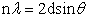(1)

Where n is the order of reflection.

lattice constants of relations estimate: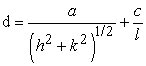(2)

Pb(Zrx,Tix-1)O3 grain size (D) can be calculated using the Scherrer equation :(3)

Where: K* is constant, θ the diffraction angle,full width half maximum.

b. Optical Properties:

Optical properties of films with different content of Zr in Pb(Zrx,Ti1-x)O3 deposited on the glass at different room temperature (RT) at a wavelength of about 0.2 to 1.1 micrometers using UV / VIS Centra 5 spectrometer that previous GBC Scientific Equipment PTY LTD was. The spectrometer consists of two deuteriums and tungsten lamp light source at a wavelength of 190-390 nm and 390-1100 nm range of the spectrum. Data output wavelength, transmission and absorption in a computer program used to deduce the optical energy gap and the underlying edge optical and optical constant.

Fixed optical parameters are very important because they describe the optical behavior of materials. Energy absorption coefficient of a material is a function of photon energy band gap is very strong. Indicates a weakening of the incident photon energy absorption coefficient per unit thickness passing through a material. The main reason for this attenuation processes of absorption attributed [11, 14]. Optical constants of refractive index (n), extinction coefficient (K), and real (εr), and imaginary components (εi) of the dielectric constant. The complex refractive index (nc) is defined as :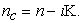(4)

It is related to the velocity of propagation (v), and light velocity(c) By: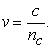(5)

The refractive index can be calculated by formula :(6)

where R is the reflectance, and can be expressed by the relation :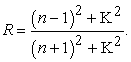(7)

Extinction coefficient that corresponds to the exponential decay of the wave as it passes through the material, as defined :(8)

(α) is given by: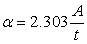(9)

Where A is the absorbance and t is the thickness of the film. The real and imaginary parts of the optical dielectric constant can be calculated using the following equation :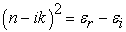(10)

where(11)

and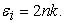(12)

c. Electrical properties:

Therefor the fundamental properties of semiconductor which determine its characteristics in addition to the width of energy gap, the mobilities of its charge carriers over a wide range of temperature. Thus the electrical properties of semiconductors are primarily interested and are dependent on the availability of holes in the valence band and electrons in the conduction band to facilitate the flow of charge under applied potential. Transport properties such as d.c electrical conductivity (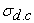), Hall effect will be discussed in this section.

The resistivity (ρ) of the film is calculated using the following equation: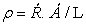(13)

Where Ŕ is the sample resistance, Á is the cross section area of the films and L is the distance between the electrodes. The conductivity () of the film was determined from the relationship:(14)

The activation energy (Ea) can be calculated from the plot of lnσ versus 1000/T according to equation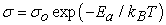(15)

Where o is the minimum electrical conductivity at 0K, T is the temperature and kB are the Boltzmann’s constant .

Hall is used to measure the semiconductor carrier concentration, mobility and it is used to detect whether a semiconductor is n or p- type.

When a constant current (I) below comes along x- axis from right to left in the presence of magnetic field(B) at z-axis with value (0.55T), the electron obeys Lorentz force in the beginning, and they drift toward the negative y- axis, and thus the sample surface charge too much and causing a transverse voltage. The Hall coefficient (RH) can be determined by measuring the Hall voltage (VH) that produces venues across the field by :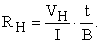(16)

From Hall coefficient equation, the semiconductor carrier concentration can be determined as given in the following:(17)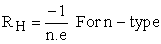(18)

Where (e) is the charge of the electron. P and n is the carriers concentrations of holes and electrons respectively. If the conduction is due to one carriers type e.g. electrons:(19)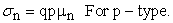(20)

We can measure the Hall Mobility as: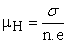(21)

or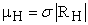(22)

i.e., by knowing σ, the mobility can be determined.

### 3. Results and Discussion

a. Structural Measurements:

Figure 1 shows the XRD patterns obtained for Pb(Zrx,Ti1-x)O3 thin films deposited on a glass substrate at RT and different concentration of (x= 0.1, 0.3, 0.5, 0.7, 0.9 wt. %. According to the International Center for Diffraction Data (ICDD), the structure of the thin films shows a polycrystalline tetragonal structure. It is obvious in the case of x= 0.1 and 0.3 reveals main peak along (101) which corresponding to 2θ = 31.5541° and a small peaks along (100), (111) and (200) corresponding to 2θ = 22.6351°, 29.2568° and 46.3514° respectively, the perovskite phase is the dominant with a small impurity phases are formed. This impurity phases are decreased and the structure exhibit's more crystallization to be complete perovskite at x = 0.5 gain sharp intensity peak, at x=0.7 the reflections (100) and (001) disappear and appear (110) reflection corresponding to 2θ = 30.8784° which indicate the change from tetragonal phase to rhombohydral phase . Rhombohydral phase still the dominate perovskite structure at x = 0.7 and 0.9 with strong reflection (101) and a two weak reflections (110) and (201).

There are some shift in the peaks resulting from substituting Zr–ions instead of Ti-ions which is larger than Ti due to the variation of distance between the internal plane d . using a computer program to calculate d-value from experimental work dexp. and compare it with the standard values as given in ICDD card dstd. and find grain size by using Scherer's formula as shown in Table 1. After a simple comparison between dexp. and dstd., it is found that the values of two parameter are identical which almost keep constant at light substitution of Zr x = 0.1, 0.3, 0.5 and gradually decrease at high substitution x = 0.7, 0.9.

#### Table 1. Shows the peaks and its Bragg's angle, interplanar distance, and full width half at maximum for Pb(Zrx,Ti1-x)O3 thin films at room temperatureDownload asVeiw figureFigures index
Figure 1. X-ray diffraction for Pb(Zrx,Ti1-x)O3 thin films with various value of x (x= 0.1, 0.3, 0.5, 0.7, 0.9) at room temperature

b. Optical Measurements:

The transmittance spectrum as a function of wavelength in the range of (300-1100)nm of Pb(Zrx,Ti1-x)O3 thin films deposited at room temperature with different Zr content (x=0.1,0.3,0.5,0.7,0.9). It is obvious from these figures and Table 2 that the transmittance is inversely proportional to concentration and that transmission increases with increasing of λ for all samples. Transmission decreases with increasing Zr content in Pb(Zrx,Ti1-x)O3. It's clear that Zr content effect on the values of transmission. Also we can observe from Figure 2 that the transmission values are decrease with increasing of Zr content in Pb(Zrx,Ti1-x)O3 may be owing to increase in thickness of the film which cause the increase in absorption. In the other hand the decreasing of the transmission may be attributed to the creation of levels at the energy band by increasing thickness and this leads to the shift of peak to smaller energies, and this results agree with Khorsand . Increasing Zr leads to increase the ratio of the large atoms resulting increase in thickness of samples and thereby increase in absorption which is in agreement with Puustinen .

Figure 3 show the absorption coefficient (α) as a function of wavelength (λ) for Pb(Zrx,Ti1-x)O3 thin films. It can be observed from the figures that the values of absorption coefficient is higher (α >104)cm-¹, this is support to expect a direct electronic transition occurs in these region . It is obvious that the absorption coefficient of the Pb(Zrx,Ti1-x)O3 films have a strong absorption coefficient at the shorter wavelength region and the absorption coefficient decrease at the long wavelength side. generally, absorption coefficient increase with the increasing of Zr concentration in the samples as shown in Table 2 and Figure 3.

The energy band gap decreases with increasing of Zr content in Pb(Zrx,Ti1-x)O3 as shown in Figure 4 and Table 2. Eg decreases from (3.41 to 3.21) eV with increasing the zr content from (0.1 to 0.9) for RT, This decreseing of Eg with increasing of Zr content is attributed to the increase of the density of the localized states in Eg which result from lack in thickness of the membranes cause a shift to lower values of Eg for different content of Zr in Pb(Zrx,Ti1-x)O3.

Figure 5 show the refractive index increases with increasing the concentration of Zr. This behavior may be happen due to the increment in the energy gap which cause expansion of the bond length in the lattice and decreases the defect which means decreasing of the reflection where the refractive index depends on it. The refractive values are increase from (2.33 to 2.64) at RT, this values are in agreement with Moret  and puustinen .

Figure 6 reveal the behavior of k is approximately similar to the corresponding absorption coefficient. It can be observe from this figure that the extinction coefficient increase with the increasing of Zr content in Pb(Zrx,Ti1-x)O3 due to increment in thickness of the films.

The real and imaginary dielectric constants of thin films were plotted with different Zr concentration in Pb(Zrx,Ti1-x)O3 at RT as shown in Figure 7 and Figure 8 respectively. (Ɛr) behavior is similar to the refractive index of less value because of k2 proportional to n2, while (Ɛi) is mainly dependent on the value of k. The dielectric constants (Ɛr) and (Ɛi) are directly proportional to the Zr content in Pb(Zrx,Ti1-x)O3 films as shown in Table 2.Download asVeiw figureFigures index
Figure 2. Transmittance spectrum as a function of wavelength for Pb(Zrx,Ti1-x)O3 films at RT and different concentrations of ZrDownload asVeiw figureFigures index
Figure 3. Absorption Coefficient as a function of wavelength for Pb(Zrx,Ti1-x)O3 films at RT and different concentrations of ZrDownload asVeiw figureFigures index
Figure 4. (αhv)2 as a function of hv for Pb(Zrx,Ti1-x)O3 films at RT and different concentration of Zr.Download asVeiw figureFigures index
Figure 5. Refractive index as a function of wavelength for Pb(Zrx,Ti1-x)O3 films at RT and different concentrations of Zr.Download asVeiw figureFigures index
Figure 6. Extinction Coefficient as a function of wavelength for Pb(Zrx,Ti1-x)O3 films at RT and different concentrations of ZrDownload asVeiw figureFigures index
Figure 7. The variation of Ɛr with wavelength for Pb(Zrx,Ti1-x)O3 films with different concentrations at RTDownload asVeiw figureFigures index
Figure 8. The variation of Ɛi with wavelength for Pb(Zrx,Ti1-x)O3 films with different concentrations at RT

#### Table 2. Illustrates the transmition, absorption coefficient, energy band gap and optical constant at RT and different concentrations of Zr for Pb(Zrx,Ti1-x)O3 thin films

c. Electrical Measurements:

DC conductivity

It is clear from these figures that there are two activation energy Ea1, Ea2 and hence two transport mechanism were calculated using the relationship (12) of a slope tangent to logarithm conductivity ln (σ) as a function of the inverted degrees of absolute temperature (1000 / T) multiplied by the Boltzmann constant (kB) in units (eV) as shown in Figure 9. the energy gap in the scores of low-lying heat and produces a second activation energy (Ea2) at high temperatures and in which transitions charge carriers in a manner stimulus or thermal irritability . It was found that the activation energy values (Ea1,Ea2) decrease with the increasing of Zr concentration as a result of decreases in the energy gap for all samples as shown in Table 3 and Figure 10. Table 3 illustrates obviously that the d.c. conductivity (σ) increase with the increasing of concentration of Zr.Download asVeiw figureFigures index
Figure 9. Lnσ versus 1000/T for Pb(Zrx,Ti1-x)O3 films with different x-concentration at RTDownload asVeiw figureFigures index
Figure 10. Variation of Ea1 and Ea2 for Pb(Zrx,Ti1-x)O3 films with different x-concentration at RT

#### Table 3. DC activation energies, their ranges and conductivity for Pb(Zrx,Ti1-x)O3 films with different Zr content at RT

Hall Effect

The results of Hall effect reveal positive Hall coefficient (p–type charge carriers) for Pb(Zrx,Ti1-x)O3 thin films i.e. Hall voltage increases with the increase of the current. Figure 11 and Figure 12 illustrate the variation of carriers concentration, Hall mobility of Pb(Zrx,Ti1-x)O3 films with different Zr content at RT. It can be observed from these figures and Table 4 that the carriers concentration were directly proportional and the mobility is inversely proportional with the different concentration of Zr, this may be due to increase the number of valence electron which result from increase of atoms.Download asVeiw figureFigures index
Figure 11. Variation of mobility for Pb(Zrx,Ti1-x)O3 films with different x-concentration at RTDownload asVeiw figureFigures index
Figure 12. Variation of carrier concentration for Pb(Zrx,Ti1-x)O3 films with different x-concentration at RT

### 4. Conclusions

The structural, optical and electrical properties of grown Pb(Zrx,Ti1-x)O3 with different concentration deposited on glass are investigated. The samples are scanned at room temperature, they were very sensitive to the change in Zr concentration. The phase were peroviskate with a polycrystalline structure. Optical energy gap decreases from (3.41 to 3.21) eV with increasing Zr concentration from (0.1 to 0.9). There are two transport mechanisms of the charge carriers in the range of temperatures (283–473) K. It was found that the activation energy values (Ea1, Ea2) decreased with the increase of Zr concentration but the d.c. conductivity (σ) increased with the increasing of concentration of Zr. Hall measurements showed that all the films were p-type. carriers concentration were directly proportional with Zr concentration but mobility were inversely proportional with Zr concentration.

### References

  F. Švegl, B. Orel, & V. Kaučič, “Electrochromic properties of lithiated Co-oxide (LixCoO2) and Ni-oxide (LixNiO2) thin films prepared by the sol–gel route,” Sol. Energy, vol. 68, no. 6, pp. 523-540, (2000).In article  M. Morozov, “Softening and Hardening Transitions in Ferroelectric Pb(Zr,Ti)O3 Ceramics,” vol. 3368, (2005).In article  M. Prabu, “Studies of pure and doped Lead Zirconate Titanate ceramics and PLD PZT thin films”. PH.D Thesis, B.S. Abdur Rahman University, (2013).In article  J. Ma, J. Hu, Z. Li, & C.-W. Nan, “Recent progress in multiferroic magnetoelectric composites,” Adv. Mater. 23, p-1062, (2011).In article  C. Jégou, L. Michalas, T. Maroutian, G. Agnus, M. Koutsoureli, G. Papaioannou, L. Largeau, D.Troadec, A. Leuliet, P. Aubert & Ph. Lecoeur, “Temperature dependence of the conduction mechanisms through a Pb(Zr,Ti)O3 thin film,” Thin Solid Films 563, p-32, (2014).In article  G.H. Haertling, “Ferroelectric ceramics: History and technology,” J Am Ceram Soc. 82: p 797-818, (1999).In article  K. Srinivas, “Influence of nanoparticles in PZT ferroelectric material properties and their applications to memory devices,” Am. J. of Nano science and Nanotechnology; 2(3) p-56-62, (2014).In article  K. Tahmasebi, & A. Barzegar, “Multiferroic Thin Film Composite of Pb(Zr0:52Ti0:48)O3 and Co-50%Fe Alloy,” Transaction F: Nanotechnology, Vol. 17,p- 108,112,(2010).In article  C. T. Q. Nguyen, M. D. Nguyen, M. Dekkers, E. Houwman, H. N. Vu, & G. Rijnders, “Process dependence of the piezoelectric response of membrane actuators based on Pb(Zr0.45Ti0.55)O3 thin films,” Thin Solid Films, 556, p-509–514, (2014).In article  W. Zhu, W. Ren, H. Xin, P. Shi & X. Wu, “Enhanced ferroelectric properties of highly (100) oriented Pb(Zr0:52Ti0:48)O3 thick films prepared by chemical solution deposition,” J. of adv. Dielectrics, Vol. 3, No. 2, 1350011, (2013).In article  M. D. Nguyen, H. N. Vu, D. H. A Blank & G. Rijnders, “Epitaxial Pb(Zr, Ti)O3 thin films for a MEMS application,” Adv. Nat. Sci.: Nano sci. Nano technol. 2, 015005, (2011).In article  C. Kittle, “Introduction to Solid State Physics,” Eight Edit. John Wiley and Sons., (2005).In article  L.V.Azaroff, “Elements of X- Ray Cryctallography,” McGraw-Hill, Inc., (1968).In article  L. U. Jian, J. C. Hu, W. H. Uang, & Z. P. Ing, “Preparation of Thick Pb(Zr, Ti) O3 (PZT) Film by Electrostatic Spray Deposition (ESD) for Application in Micro-System Technology,” Appl. Phys., vol. 41, no. 6, pp. 4317-4320, (2002).In article  S. Kim, J. Yang, C. Y. Koo, J. Yeom, D. Lee, & J. Ha, “Electromechanical Properties of Pb (Zr, Ti) O 3 Films for MEMS Applications,” vol. 42, no. April, pp. 1101-1104, (2003).In article  P. S. Kireev, “Semiconductors physics,” 2nd edition. translated from the Russian by M. Samokhvalov, Mir puplishers Moscow, (1978).In article  A. Islam, M. Islam, M. Choudlury & M. Hassan, “Recent Velopment in Condenced Matter Physics and Nuclear Science,” no. Rajshahi University, Bangladesh, (1998).In article  T. Kamakshi & P. S. V. SubbaRao, “Softening transitions in ferroelectric Pb (Zr, Ti) o3 ceramics doped with neodymium oxide and lanthanum oxide,” J. Appl. Phys., vol. 6, p. 50-55, (2014).In article  K. Wafaa, D. Sariya & R. Sura, “Structure and Morphological Properties of Cadmium Oxide Nanostructure Prepared By Oblique Angle Deposition Method,” ICNAMA, (2015).In article  A. Khorsand & W. Majid, “Effect of solvent on structure and optical properties of PZT nanoparticles prepared by solgel method, in infrared region,” Ceram. Int., vol. 37, pp. 753-758, (2011).In article  J. Puustinen, “Pase structure and surfase morphology effects on the optical properties of nanocrystalline PZT thin films,” Universty of Oulu, (2014).In article  L. Holland & G. Siddall “the properties of some reactively sputter 4ed metal oxide films,” Vacuum, vol. 3.4, pp. 375-391, (1953).In article  M. Moret, M. Devillers & K. Worhoff, “Optical properties of PbTiO3, Pb(Zrx,Ti1-x)O3, and PbZrO3 films deposited by metalorganic chemical vapor on SrTiO3,” J. Appl. Phys., vol. 92, no. 1, pp. 468-474, (2002).In article